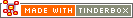# Conditional statements using multiple argumentsIf() conditional statements and queries may use more than one argument using logical joins.

( A & B )

Reads: if condition A AND condition B are true, then this compound query is true.

Contrast this with the &= operator (below).

( A &= B )

Reads: result is true if condition A is currently true and, if so, if condition B is also true.

Thus, if condition A is false, condition B is not checked and the overall result is false. Thus in some cases only condition A is evaluated.

By comparison, with a ( A & B ) query both condition A and condition B are are always evaluated.

( A | B )

Reads: if condition A OR condition B is true, then this compound query is true.

Contrast this with the |= operator (below).

( A |= B )

Reads: result is true if condition A is currently true or, if so if condition B is true.

Thus, if condition A is false, query B is not checked and the overall result is false. Thus in some cases only condition A is evaluated.

By comparison, with a ( A | B ) query both condition A and condition B are are always evaluated.

(A | B) & ( C | ( D | E ) )

This is a more complex example of logical joins. Reads: if (condition A OR condition B is true) AND (condition C OR (either condition D OR condition E are true)), then this compound query is true.

 Up: Actions & Rules Previous: Conditional Actions (if clauses) Next: Expressions in paths

[Last updated: 14 Dec 2009, using v5.0]

Google search aTbRef for:

Licensed under Creative Commons Attribution-Noncommercial-Share Alike 3.0 License
[See aTbRef CC licence Attribution/Waiver info info]